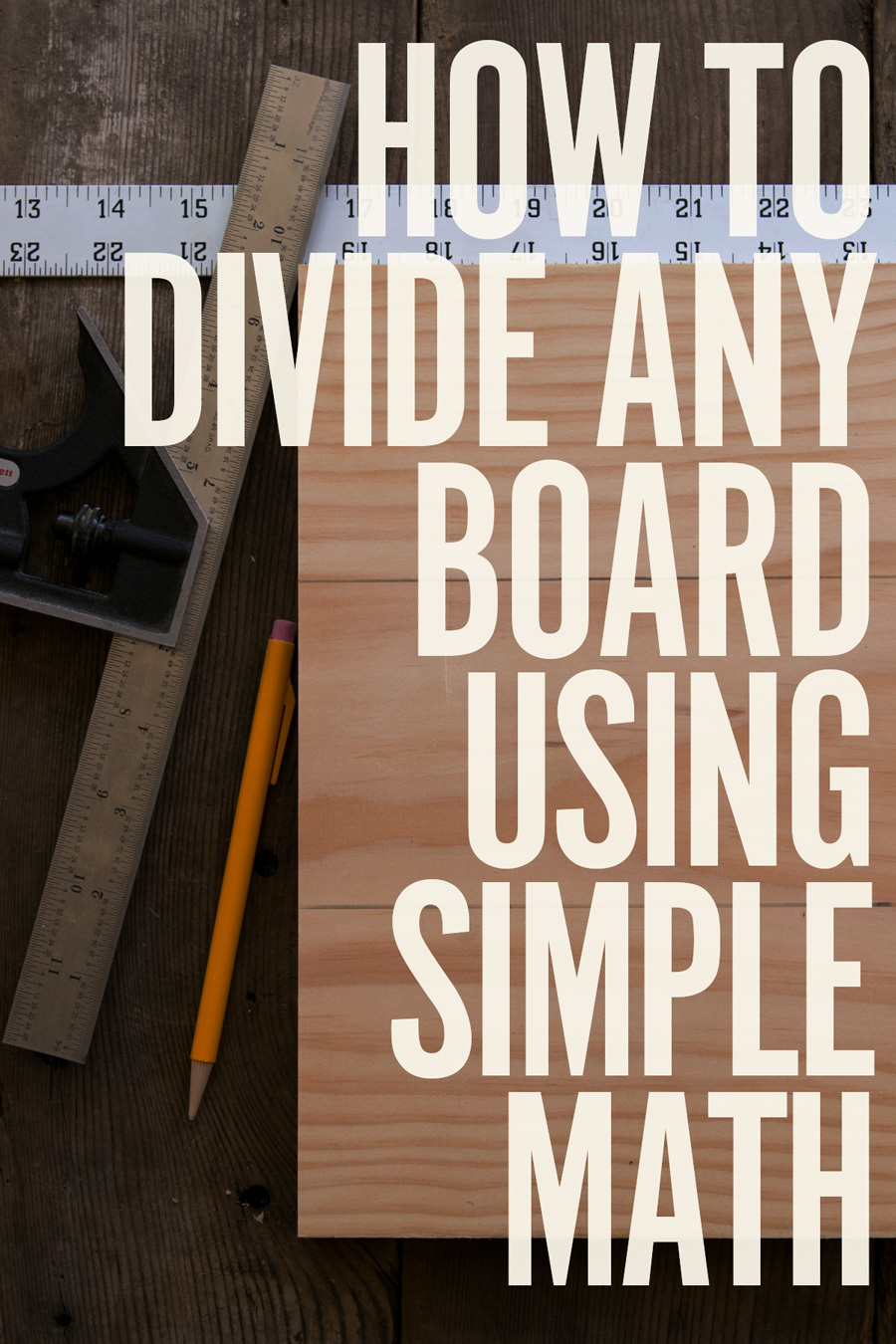# How to Divide Any Board into Equal Parts without Fractions or Complicated Math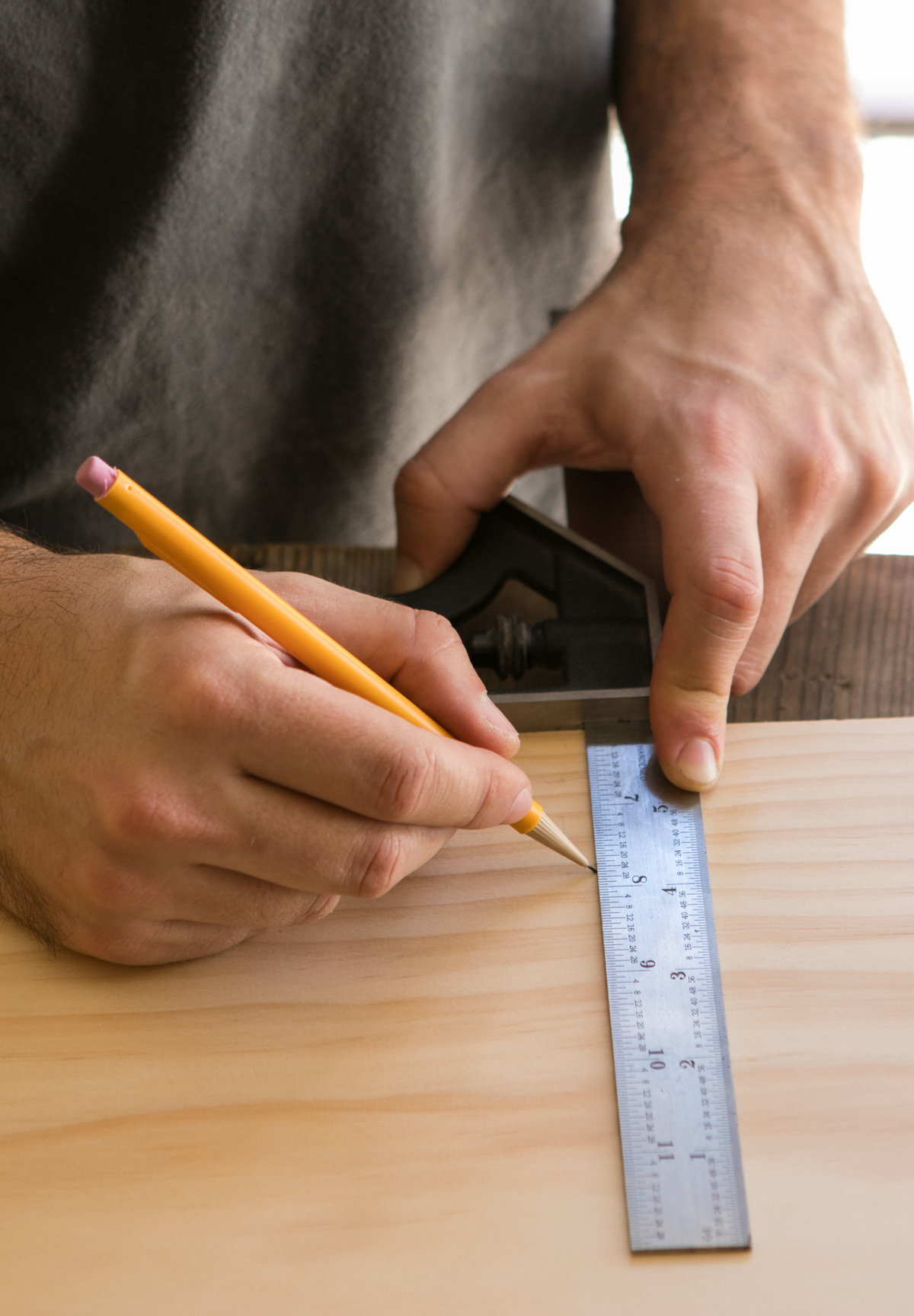If you do woodworking and DIYing in inches, a solid understanding of fractions is essential. Being able to calculate that half of 4 1/4″ is 2 1/8″, or that 1 1/2 + 1 3/16 = 2 11/16″ is basic shop math that will keep your projects moving quickly.

But often, bringing fractions into the process is, well, completely unnecessary. Let's say you have a board that you'd like to divide into equal parts. You could measure it, then bust out a pencil, paper, and the calculator app, and eventually have to Google a decimal-to-fraction converter to figure out the size of each section. Then, you'd have to find that crazy number on your ruler, and carefully add the units together to mark out your parts. Or… you could just do this.Okay, let's say this is a board from a project I'm working on. I want to divide it into three equal parts.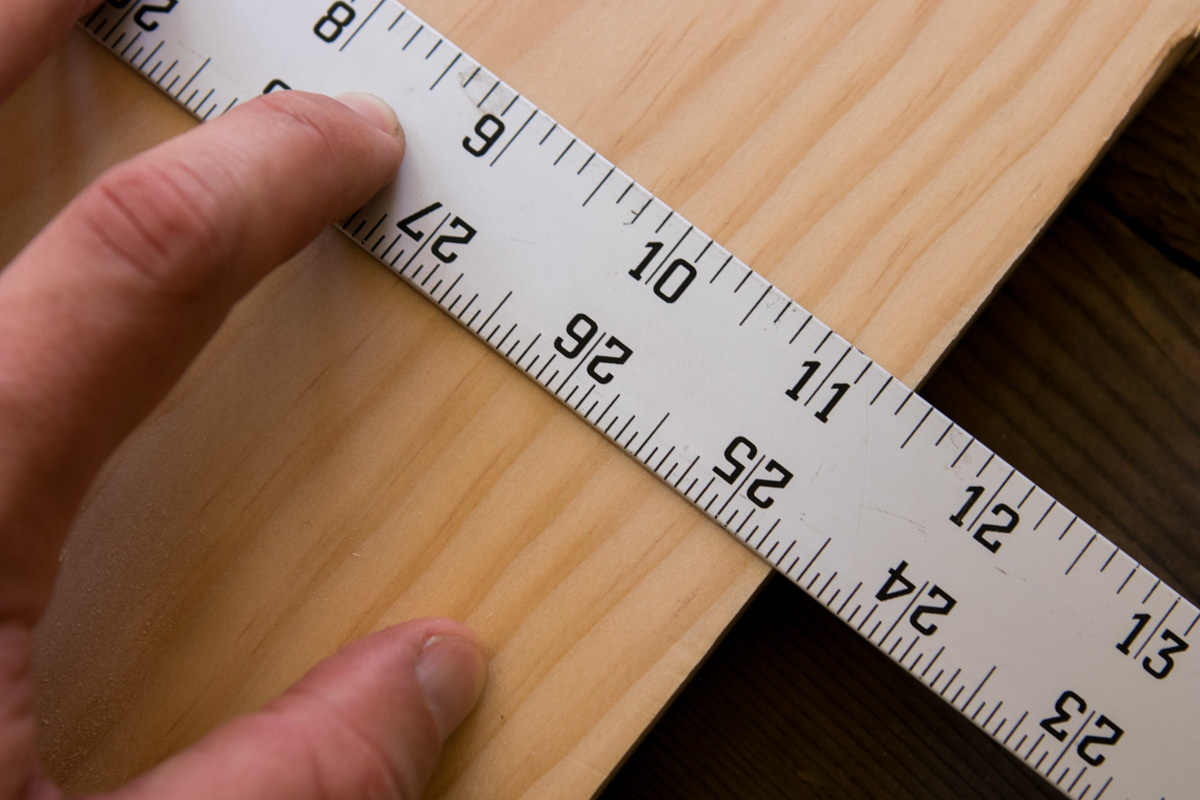The board measures 11 3/8″ wide. If I divide that number by three, I get 3.79167. In fractions, that's 3 19/24, or, in 4r ruler language, about 3 51/64″. Or something. Just thinking about that makes my head hurt.

The truth is, I don't care what that number is. I just want the width to be divided equally. So, since I can't find that number on my ruler easily, I make this simple move: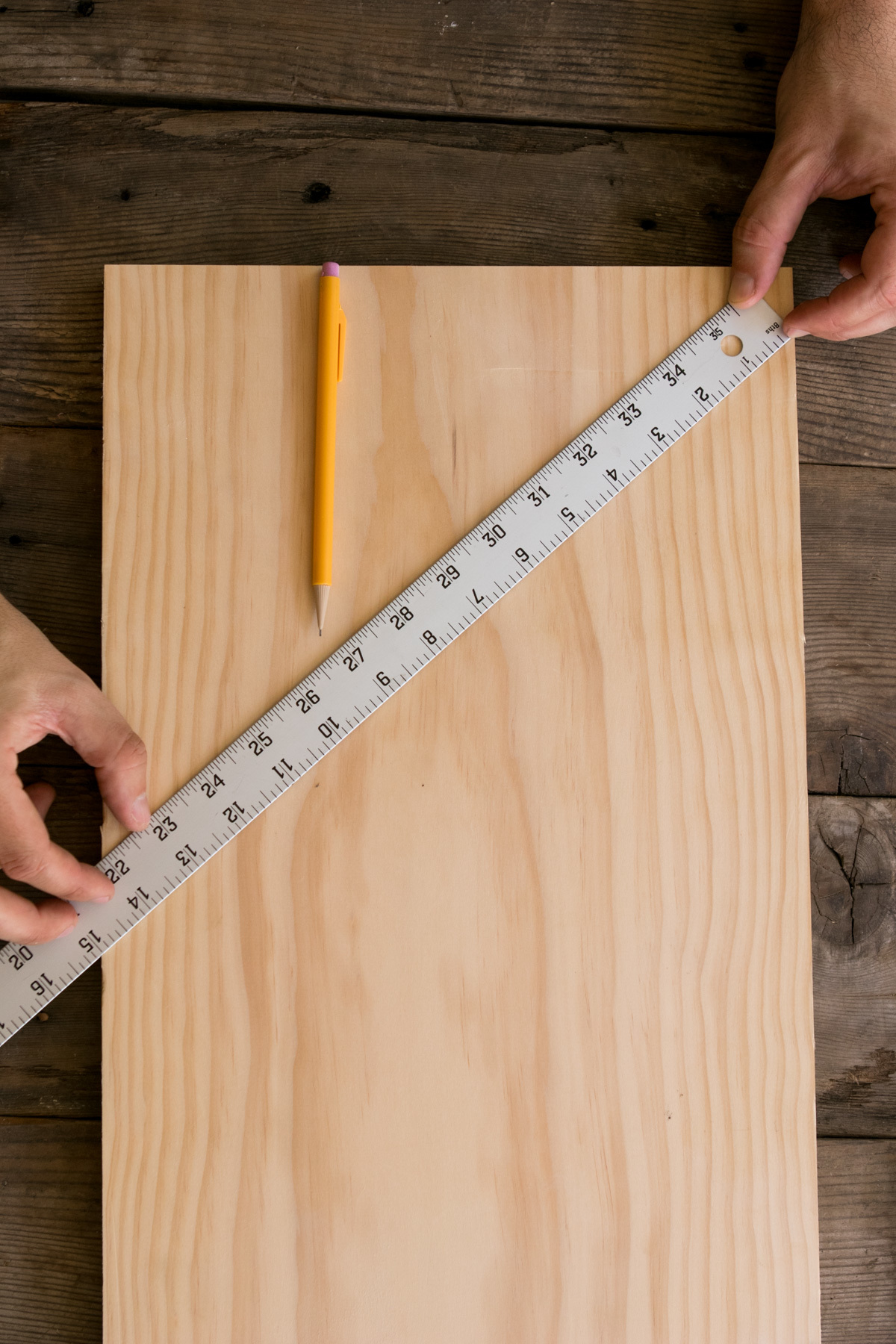I pivot the ruler until it reads a number I can divide by evenly. I'm looking for multiples of three, because that's how many sections I'm looking for. 12″ works great, as does 18″. In this case, I chose 15″.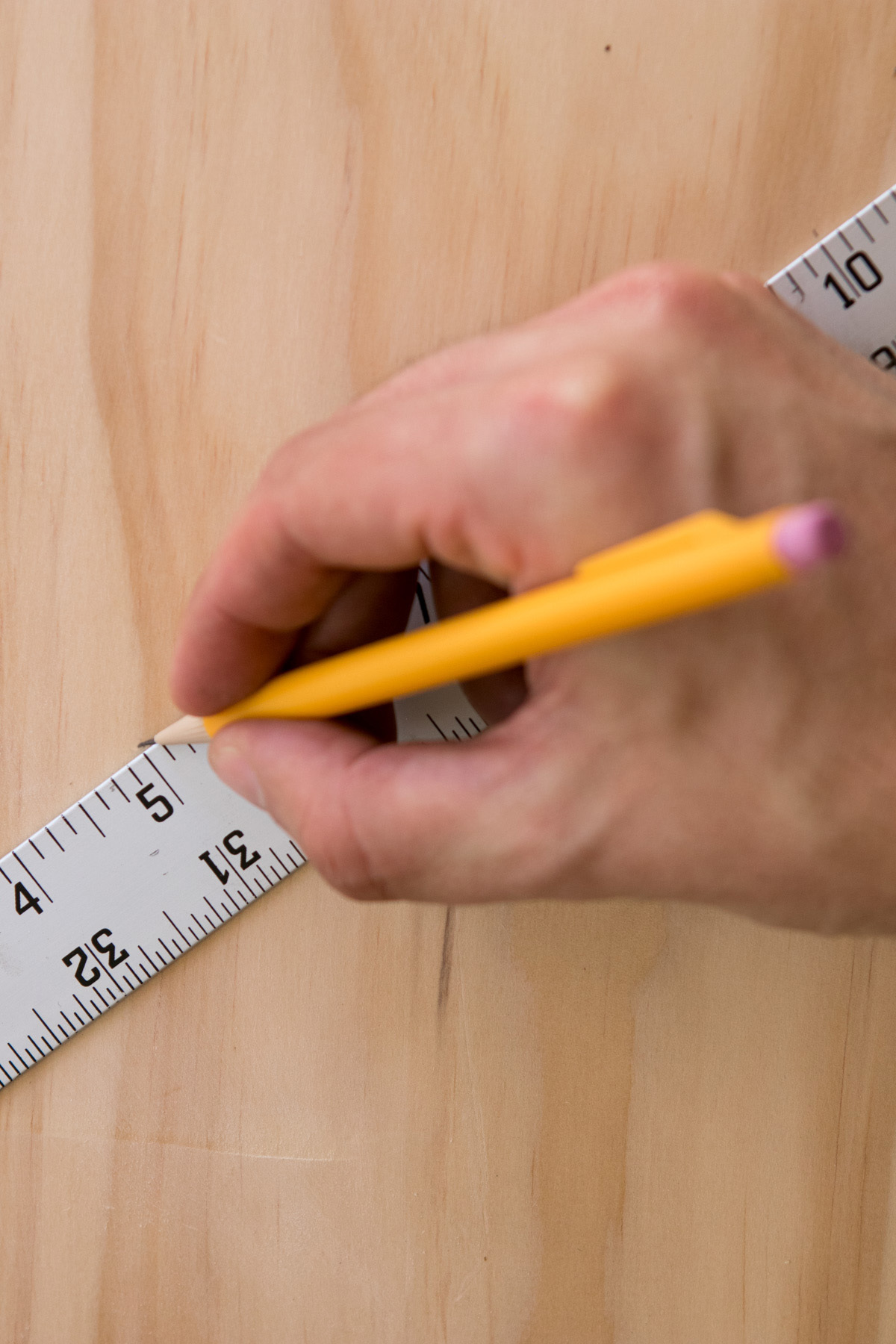Now, I just make marks at the whole numbers that represent my sections. Making sure my ruler falls exactly at 0″ and 15″ on the edges, I make a mark at 5″, and another one at 10″.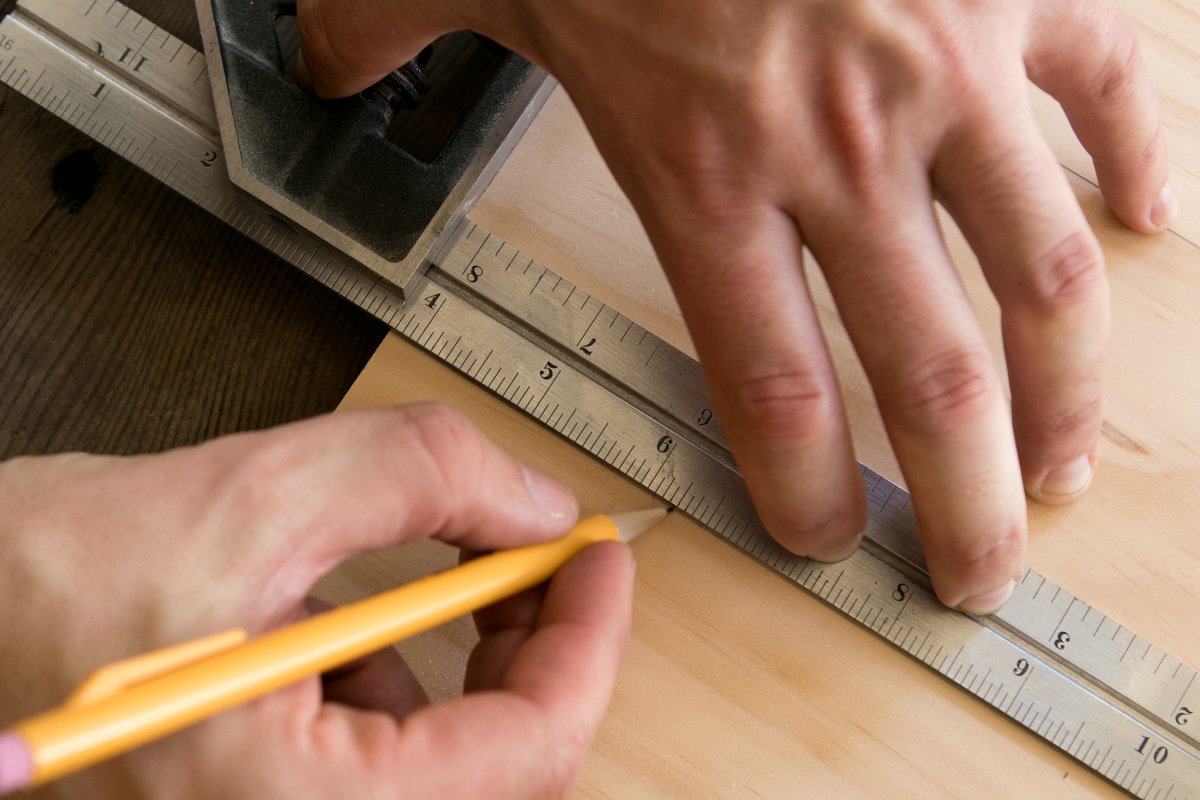Then, I use a square to extend that line to where I need it, say, for a baseline for cutting dovetails.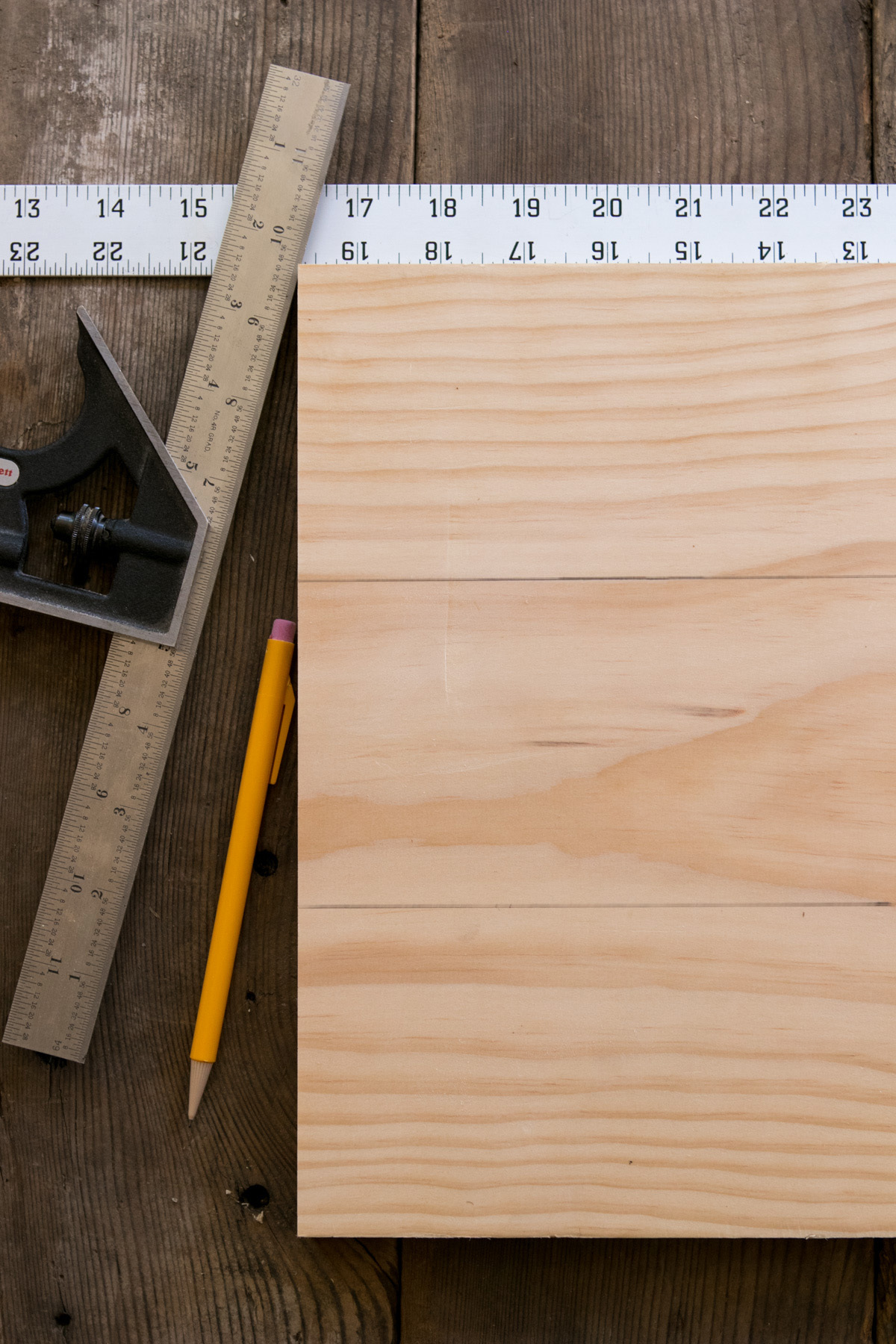### There you go. Three even sections.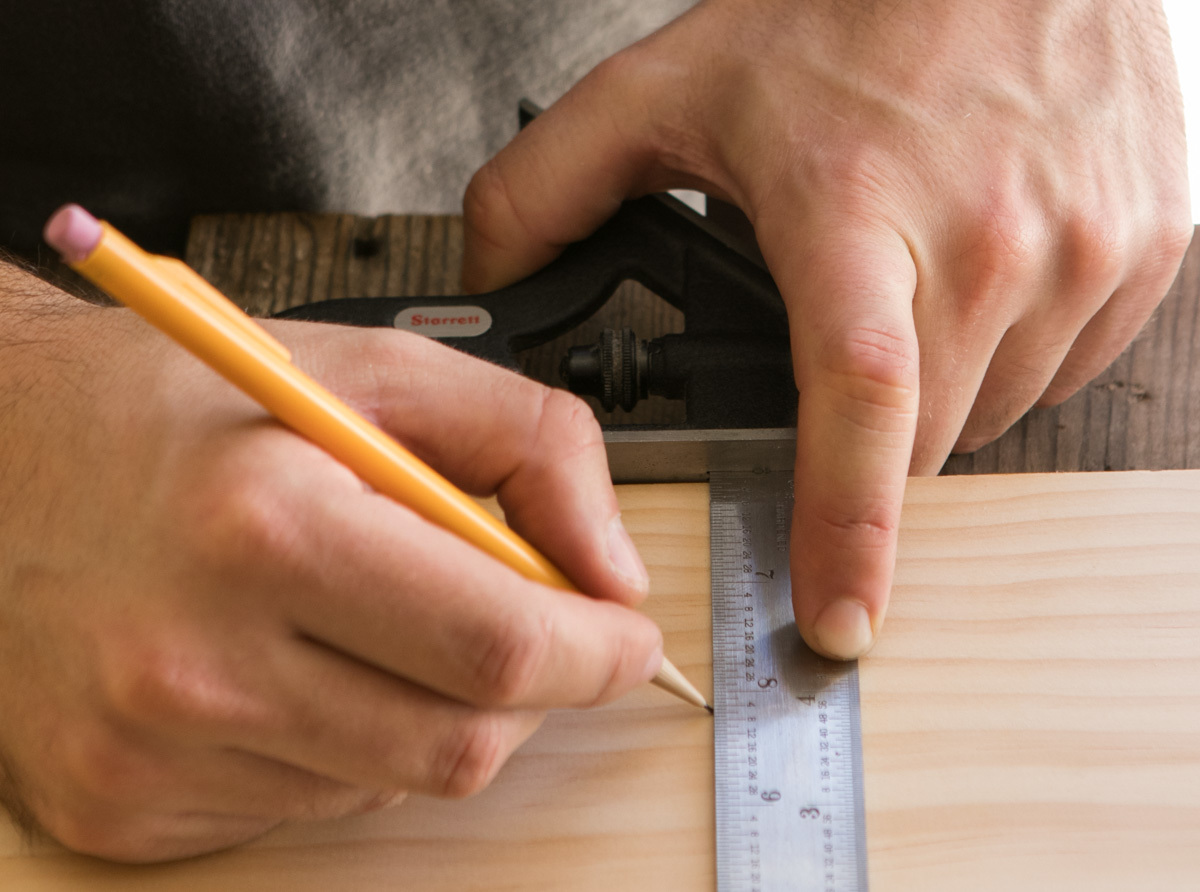Let's try another number. This time, I want four sections. So I:

1) Rotate my ruler until I find a number easily divisible by 4, such as 16″.
2) Make a mark at 4″, 8″, and 12″
3) Extend the lines with a square

If I want seven sections, I'd:

1) Rotate my ruler to 14″
2) Make a mark at 2, 4, 6, 8, 10, and 12″
3) Extend those line with a square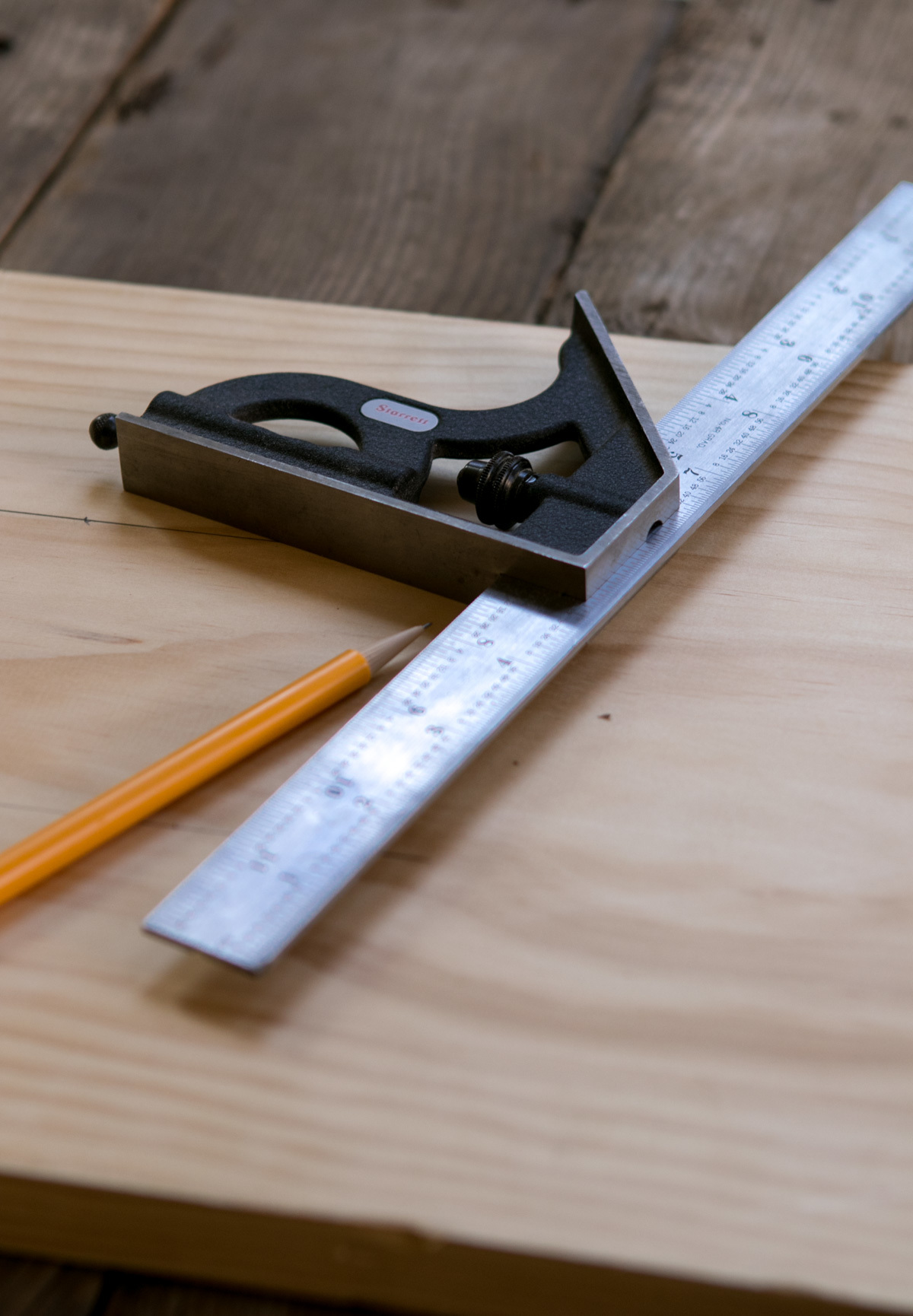## Now. Go do good work.